# Combinatorial Logic and the PLC Program

Before getting started with the PLC programming, let me illustrate some basic concepts about a PLC program.

If you know about the PLC scan time, you can start to explore how the PLC program works. Combinatorial logic gives you the basic understanding of how combining the two states – true and false with logic. The basics of PLC programming is combining logic. That’s why combinatorial logic can be a great way into PLC programming. Since the fundamentals of combinatorial logic and PLC programming are the same.

## Input logic

The PLC program can be divided into two parts. The first part controls or decides the second part. The first part is called the input logic. The input logic is the function of the PLC program. As the word says the input logic is the logic of your program. But what is logic? It is important to know what logic is, because the PLC program is logic. To be more precise the PLC program is digital logic; in mathematics called boolean algebra.

In boolean algebra or digital logic the values can be only one of two states: true or false. In PLC’s the true or false is represented by 0 for false and 1 for true. This is how microprocessors and computers work. They work with 0’s and 1’s, and when we are programming the PLC we have to think in 0’s and 1’s. We are using boolean algebra and logic when we are programming a PLC because that is how they work.

### Logic with a real-world example

Starting with a real-world example, let us find out what digital logic really is all about.

This simple example will be a light bulb and two switches. Two simple parts with one important thing in common: they both have two states. The light bulb and the switches are either on or off.

We can connect the light bulb and the switches in different ways. This will make the switches and the light bulb function in different ways.

The first way we could way we could connect the switches and the light bulb is to connect them all in one straight line from the line the the neutral. This is called connecting the switches in series:When we are connecting the switches in series before a light bulb, both switches has to be on to turn the light bulb on. In digital logic this would be called an AND-gate. Why is that? Because both the first switch AND the second switch has to be on. In our real-world example the states of the switches and light bulb are on, and off and in digital logic the two states are true and false. Think of it as the switches and the light bulb representing the states true and false. If they are on they are true and if they are off they are false.

## Combinatorial logic and the six gates

Now, let us go back to the so called AND-gate. The AND-gate is a way of deciding the state of an output with two inputs as decisions. This is called combinatorial logic because we are combining more inputs (variables in boolean algebra).

### AND-gate

All gates in combinatorial logic has inputs and outputs. The state of the output is then decided by the inputs. So with an AND-gate both inputs has to be true for the output the be true. In out real-world example the two switches are the inputs and the light bulb are the output. In combinatorial logic the state of the output are decided immediately. So, as soon as the inputs are there the state of the output will be set. If we turn on both switches the light bulb will be turned on.

The AND-gate can be drawn with a symbol like this: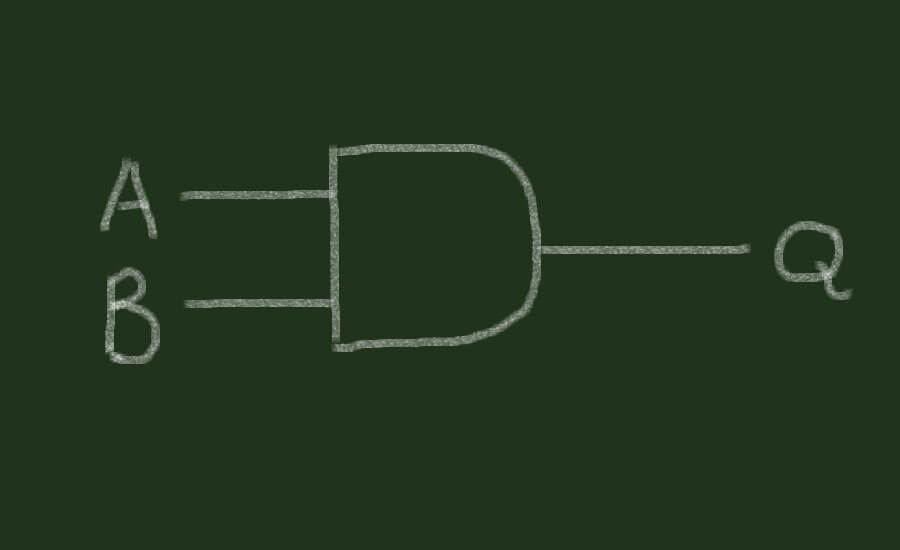The standard names or symbols for inputs in digital logic are A and B. For the output the standard symbol is Q. The gates we will be working with for now all has two inputs and one output. Each gate performs a simple function by setting the state of the output. Some gates might have more than two inputs, but all gates will only have one output.

The state of the inputs and outputs can be illustrated with a truth table. For every combinatorial logic gate we can plot a truth table to describe its functionality.

The truth table for the AND-gate will look like this:

 A • B A false true B false false false true false true

With this truth table we can see the functionality and the states of the output with every possible combination of the inputs.

The content of the large field in the upper left corner is the formula for and AND-gate. The AND-gate (A and B) should be written as: AB, A^B or A • B.

The fields marked with a thick line are the output results of the AND-gate symbolized by Q. The two fields below A and the two fields next to B are the values of the inputs A and B. The values can be false and true, but to make it more easy for us, let us change the true and false with 0’s and 1’s. In most PLC’s the status of inputs and outputs are described with a 0 for false and a 1 for true.

Here is a table to compare the different names and terminology for our real-world example, combinatorial logic, digital logic and the electronics in the PLC:

 Light switches Combinatorial logic PLC program logic PLC electronics off false 0 0 volt on true 1 5 volt

Let us take a look at the truth table for the AND-gate, but this time with the 0’s and 1’s for representing the states of the inputs and outputs:

 A • B A 0 1 B 0 0 0 1 0 1

The output Q will only be 1 (true) if both inputs A and B are 1 (true).

Now, this gate might seem simple with only two inputs and one output, but this AND-gate is actually a simple PLC program. Try to imagine that A and B are inputs on a PLC and Q is an output on the PLC. A PLC program is just one or more logic gates. This will be explained in the next chapter, when we start the actual programming of PLC’s. The important thing to understand now, is that a PLC program is a piece of logic – just like the AND-gate. The PLC program takes the states of the inputs (just like the gate) as decisions for the state of the output (just like the gate).

## More logic gates

### OR-gate

The AND-gate is not the only type of gate in combinatorial logic. If we move back to our real-world example with the two switches and the light bulb, we can connect them in a different way. The two switches can be connected in “parallel” to each other before the light bulbs. This will make the switches work in a different way. Let us take a look at the schematic for this “parallel” connection:We call this type of connection a parallel connection because the two switches are parallel to each other. The parallel connection works a little different than the serial connection we used before. Now, that each switch has direct contact to the light bulb, each switch will turn on the light bulb. So to turn on the light bulb either the first switch OR the second switch has to be on. This is the functionality of an OR-gate.

The symbol for the OR-gate looks like this: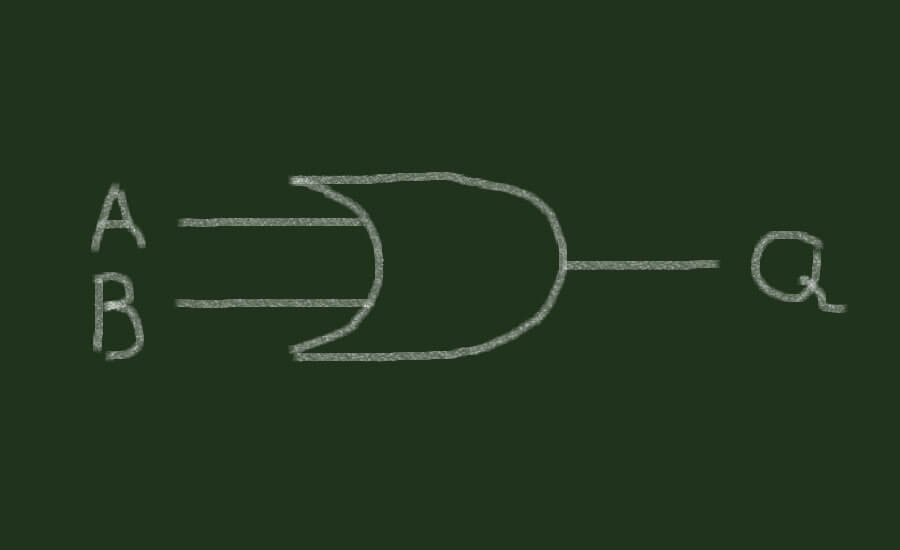Just like the AND-gate we learned about before, the OR-gate has two inputs and one output. The symbols for the inputs are A and B and the symbol for the output is Q.

Since the OR-gate work a little different than the AND-gate we can plot a new truth table for the OR-gate:

 A + B A 0 1 B 0 0 1 1 1 1

As we can see in the fields outlined by thick lines, the output of the OR-gate will be 1 (true) if either input A or input B are 1 (true).

The formula for the OR-gate is written as: A + B or A∨B.

Both the AND-gate and the OR-gate are simple logic gates, because they are relatively simple to explain with two switches and a light bulb. The two switches representing the inputs (A and B) and the light bulb representing the output (Q).

### NOT-gate – The inverter

In combinatorial logic there are 5 gates we need to know about, so with three left let us move on to the next gate: the NOT-gate. With the AND-gate and OR-gate, these three gates are the basic gates in combinatorial logic. From these gates we can derive the other two gates, but first let us take a closer look at the NOT-gate:NOT-gates are special because the output are the same as the input. The input is just inverted to the opposite state. That is why it is called a NOT-gate. Because when the input is true the “output” will NOT be true, but false.

The NOT-gate is also called an inverter because it takes the value of the input (A) and inverts it to the opposite state. This can be illustrated with the truth table for the NOT-gate. You might notice that the truth table for the NOT-gate looks a little different than the previous two truth tables we have looked at so far:

 A A 0 1 1 0

The values in the first column below the A are the states of the input (A) and the values in the second column below the A are the “output” values of the NOT-gate. But since the input and the output are the same we can’t really talk about outputs with this gate. The NOT-gate is really not a gate, because it does not make any decisions. It only changes the value of one input.

The formula for the NOT-gate is written like this: A, A’, ¬A and sometimes NOT A or !A.

## Derived logic gates

With the three gates that we now know about, we derive the last two gates. The last two gates are a little more complicated. To explain them, in terms of our real-world example with the switches and the light bulb, will require us to expand the functionality of the two switches. The next two gates works a little different than the first two gates, so we cannot use the simple example with just two switches. If we take a look at the next gate, the XOR-gate, that will be clear.

### XOR-gate

The XOR-gate is also called the exclusive OR-gate, because its functionality looks a bit like the OR-gate but with one crucial difference. When both inputs are true in an OR-gate the output will be true. But when both inputs are true in a XOR-gate the output will be false.

First, have a look at the truth table for the XOR-gate:

 A ⊕ B A 0 1 B 0 0 1 1 1 0

Notice the underlined 0 in the lower right corner of the table. That is the difference between the OR-gate and the XOR-gate.

The formula for the XOR-gate is written as following: A ⊕ B.

Before moving on to our real-world example, let’s try to build the XOR-gate with the three basic gates in combinatorial logic. Remember that the last two gates including this XOR-gate can be derived (build) with the basic three gates.

The XOR-gate can be build or derived by using two NOT-gates two AND-gates and one OR-gate: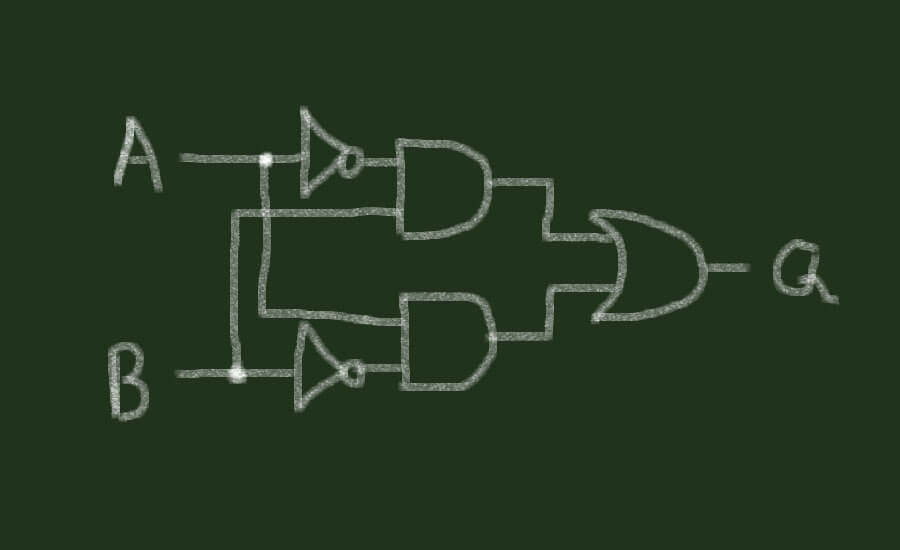The functionality of the five gates combined are equivalent to the XOR-gate. The output will be 1 (true) if either input A or input B is 1 (true), but not if both inputs are 1 (true).

### Normally open and normally closed

Moving back to our real-world example we can now expand the functionality of the two switches.

Switches can work in two different ways: by making a connection or by breaking a connection. These two ways of functioning are called normally open and normally closed. The names are defined by the default state of the contacts in the switch. If the contact is “open” and does not make a connection it is called a normally open contact or a NO-contact. The normally open contact is drawn like this:The other contact is called the normally closed contact, because the default state of the contact makes a connection. The normally closed contact or the NC-contact is drawn with this symbol:A switch can have more than one contact. It is not unusual to have two contacts in a switch, where one of them is normally open and the other is normally closed. This gives the switch two functions: a make and a break function.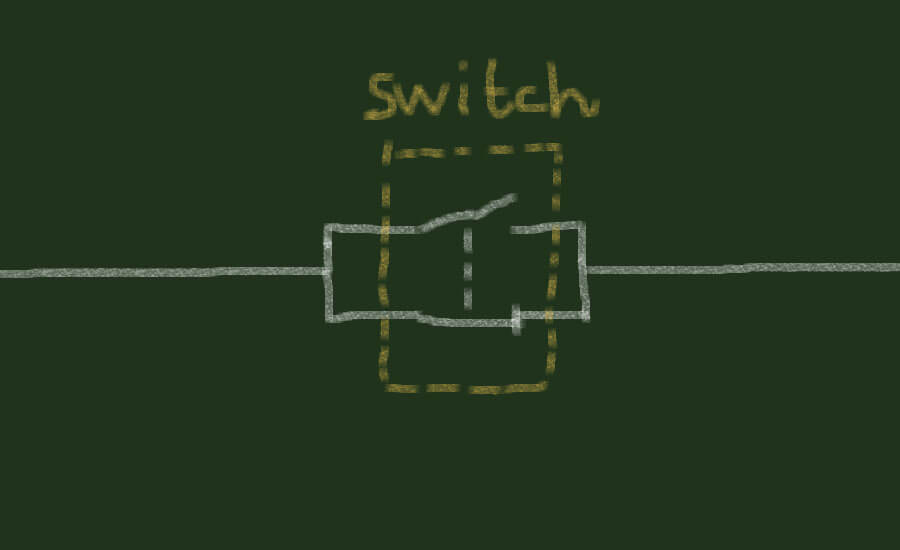When the switch above is activated the NO-contact will make a connection and the NC-contact will break or disconnect the connection. All the contacts inside the switch will be activated at the same time, when the switch is activated.

To illustrate the first two gates, the OR-gate and the AND-gate we used normally open contacts in our switches. This meant that when we activated the switches a connection would be made. But to illustrate the XOR-gate with our real-world example we have to use NC-contacts too. Why? Because the NC-contacts are the same as the NOT-gate.

Think about it. The NOT-gate inverts the input signal and the same is true with the NC-contact. If we plot the truth table for the NC-contact this will be more clear:

 NC – IN NC – OUT OFF – 0 _.__._ ON – 1 ON – 1 _./ ._ OFF – 0

This truth table looks just like the truth table of the NOT-gate. When the NC-contact is off or in its default state the contact will make the connection and the output of the contact will be on or 1 (true). And if the NC-contact is activated or in the state of true or 1 the output will be 0 or false. The normally closed contact is doing the same job as the NOT-gate: inverting the signal to the opposite state.

This gives us three rules to follow:

• Serial contacts are the same as AND-gates.
• Parallel contacts are the same as OR-gates.
• NC-contacts are the same as NOT-gates.

So, how can we connect our switches and light bulb to illustrate the XOR-gate? First we need to take a look at the connections we made for the OR-gate:This works just fine, but remember the difference between the OR-gate and the XOR-gate? When both contacts are activated in the OR-gate the light bulb is on, but with the XOR-gate the light bulb has to be off. So, in order to build the XOR-gate we need to prevent the light bulb from turning on when both contacts are activated.

Remembering that the OR-gate is equivalent to a parallel circuit, let us try to make use of a normally closed contact in one of the switches. This new contact can be connected to our parallel connection in different ways. First we could connect it parallel to the other two contacts:This would not make any sense though, since the light bulb would now be turned on all the time. Take a look at the truth table:

 Switches A and B A OFF ON B OFF ON ON ON ON ON

No matter if the switches are on or off the light bulb will always be turned on.

Since that did not work, let us try to connect out new NC-contact in a different way: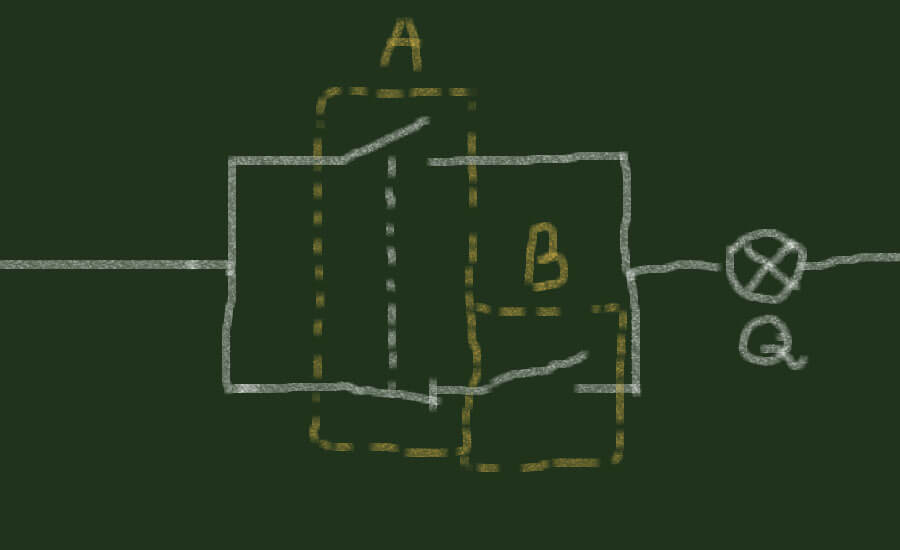Now, the first switch has two functions or two contacts. One for making a connection and one for breaking a connection. If we take a look at the truth table for our new circuit between the contacts in the switches and the light bulb, you might notice that the functionality is the same as with the OR-gate:

 Switches A and B A OFF ON B OFF OFF ON ON ON ON

The new NC-contact did not make any difference. But what if both switches had two functions. Next step will be to use both the normally open and the normally closed contacts in the two switches.

But this time we will be using the three rules listed above. We need to use the rules, because we can connect the contacts and the light bulb in so many different ways. We could of course just try to guess how we should connect the contacts to make an XOR-gate. But when we are following the rules it should take less time to derive the XOR-gate.

Since we already have an OR-gate we can apply the first rule: the parallel connection is the OR-gate. We already have the parallel connection with the normally open contacts, so this would make up the OR-gate. But since the output has to be NOT true if both the switches (inputs) are activated this might tell us that we should use some NOT-gates. This is the second rule we will be using: the NOT-gates are the normally closed contacts. We need to insert some NC-contacts in out real-world example.

If both switches are activated the light bulb should not be activated, so we might insert a normally closed contact from each switch in our example.

What we now have is an OR-gate, and what we need is to break both connections to the light bulb when both switches are activated. We have to break both connections or inputs to the light bulb, because the if just one of the inputs in an OR-gate is true the gate will be true. This can be done by connecting the normally closed contacts of the switches in serial connection the the normally open contact on the other switch: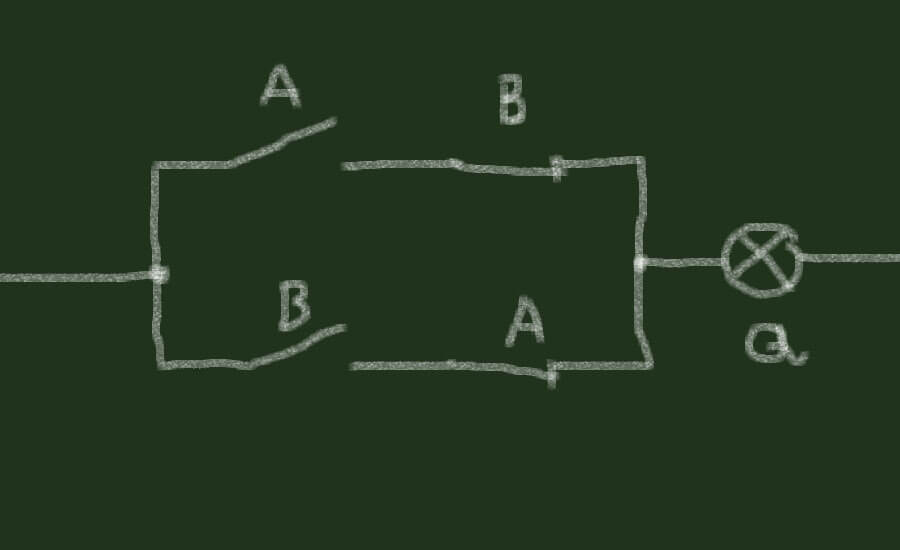Looking at the truth table for this circuit, and we will see that we have now build an XOR-gate. It works just like the OR-gate, but with the one difference that when both switches are activated the light bulb will not be activated.

 A ⊕ B A 0 1 B 0 0 1 1 1 0

With four gates explained we now have only two gates left. The last two gates are the opposite of the first two gates or the inverted versions of the first two gates. This means that the output will be the opposite or inverted value with the same input state as the first two. The last two gates are called the NOR-gate and the NAND-gate.

### NOR-gate

The NOR-gate and the NAND-gate is the opposites of the OR-gate and the AND-gate. They are the inverted ones because, the “N” we put in front of the names stand for NOT. The NOR-gate is the NOT OR-gate and the NAND-gate is the NOT AND-gate.

First, take a look at the truth table for the NOR-gate. The NOR-gate is the inverted OR-gate:

 A NOR B A 0 1 B 0 1 0 1 0 0

If you look at the output states marked with thick lines, they are all the exact opposites as with the OR-gate. This is why it is called the NOT OR-gate. When we are deriving or building the NOR-gate with the three basic gates, the name also makes sense. The NOR-gate is derived from the OR-gate and the NOT-gate. The NOT-gate is inverting the output of the OR-gate which gives us the inverted OR-gate also known as the NOR-gate.The same principle of deriving the opposite gate happens when deriving the last of the six gates in combinatorial logic – the NAND-gate.

### NAND-gate

A NAND-gate is the opposite of the AND-gate and is also called the NOT AND-gate. The NAND-gate is derived from the AND-gate and the NOT-gate in the same way as the NOR-gate. The NOT-gate inverts the output of the AND-gate which makes the opposite of the AND-gate – the NAND-gate.

Both the NAND-gate and the NOR-gate can be illustrated in out real world example with the two switches representing the inputs and the light bulb representing the output. To do that, we need to take a look at the truth tables.

Here is the truth table for the NAND-gate:

 A NAND B A 0 1 B 0 1 1 1 1 0

As you can see in the truth table, the output is always 1 (true) except when both inputs are 1 (true). This means that even when both inputs are 0 (false) the output will be 1 (true). With this in mind we now know that we have to use the normally closed (NC) contacts in our switches. They will turn the light bulb on (true) when they are off (false).

Now comes an interesting thing that leads to a more advanced part of combinatorial logic or boolean algebra. Remember that, one of the rules said that a parallel connection is an OR-gate. This is only true when the inputs are not inverted. Because, when we are illustrating the NAND-gate with the switches, the two switches are connected parallel to each other: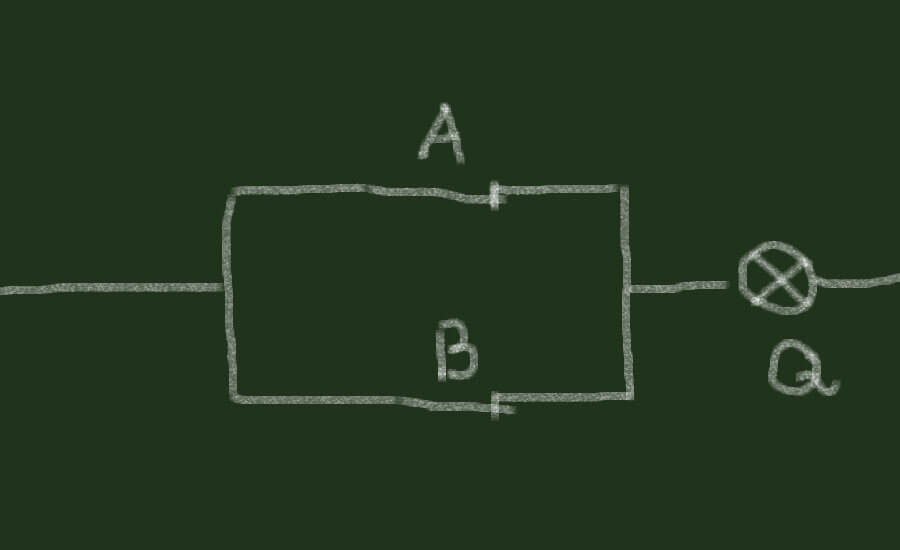When we are inverting the inputs (in our example: using normally closed instead of normally open contacts), the parallel connection makes a NAND-gate. The description of what happens when the inputs are inverted are called DeMorgan’s Theorem.

DeMorgan’s Theorem also applies when we are illustrating the NOR-gate. The NOR-gate in our example would be the two switches in serial connection before the light bulb. Since the NOR-gate is an inverted gate we would use normally closed contacts. This also breaks one of the rules. The rule that says serial connections are AND-gates. Serial connection are only and gates when we are using normally open contacts. When the contacts are normally closed, illustrating that the inputs are inverted, the serial connection will be the NOR-gate.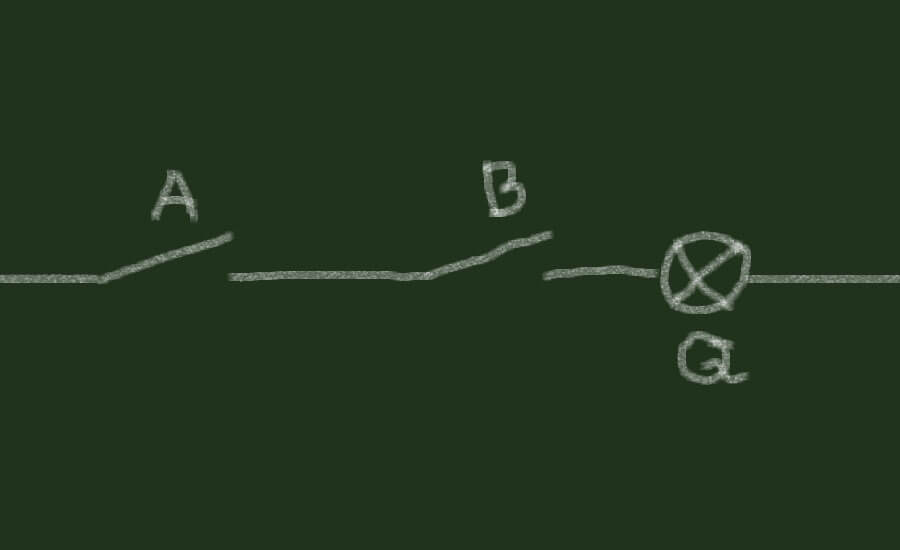The easiest way to derive the NOR-gate and the NAND-gate is to invert the inputs. Illustrated in our example as using normally closed contacts representing the inverted inputs. But when we derived the NOR-gate and the NAND-gate using the basic gates we were inverting the output and not the inputs.

Let us try to derive the NOR-gate by inverting the inputs of an OR-gate: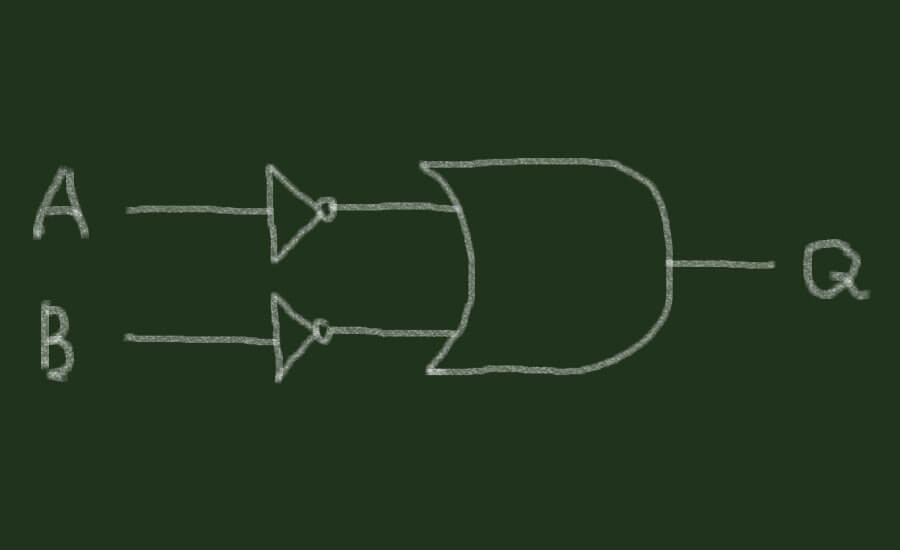This would have the same truth table as if we inverted the output of an OR-gate. Exactly the same would happen if we inverted the input signals of an AND-gate to make a NAND-gate. From these observations we can make another rule:

Inverting the inputs or inverting the output has the same effect on the functionality.

At last, let us try to invert the outputs in our real-world example and see if we can illustrate the NAND-gate and the NOR-gate. In our example the light bulb is representing the output. This means that we would have to invert the function of the light bulb. But how?

The answer is relays. Relays are a set of contacts build together in a small container. The relay is activated by electricity, just like the light bulb. But when the relay is activated all its contacts are activated too. The relay is just like our switches, but instead of manually the contacts are activated electrically.

To invert the output we have to put in a relay instead of the light bulb. Now the output will activate the relay instead of the light bulb:To invert the output we still need the light bulb, so by making a new connection between the light bulb and one of the contacts on the relay, we can now activate the light bulb with the relay. But to make the relay activate the light bulb in the opposite way (inverted output) we have to use a normally closed contact in the relay. This will invert the output and thereby our light bulb:This inverting of the output in a parallel connection will give us the NAND-gate. We could invert the output of the serial connection with a relay as well and get the NOR-gate.

With all the gates illustrated, we now have a basic knowledge of the six gates in combinatorial logic:

• AND-gate
• OR-gate
• NOT-gate
• XOR-gate
• NAND-gate
• NOR-gate

Knowing about gates and digital logic is vital for learning PLC programming.

If you would like to build logic circuits and learn here are two great resources you can use:

• You can use The Logic Lab to simulate all the gates with inputs and outputs.
• You can also download Logisim, a logic circuit simulator used by universities and students for education.
•